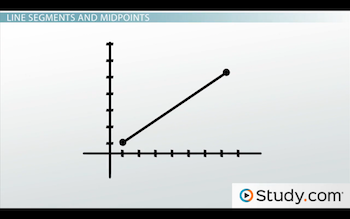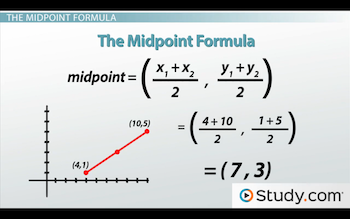# How to Use The Midpoint Formula

An error occurred trying to load this video.

Try refreshing the page, or contact customer support.

Coming up next: How to Use The Distance Formula

### You're on a roll. Keep up the good work!

Replay
Your next lesson will play in 10 seconds
• 0:05 Line Segments and Midpoints
• 1:18 The Midpoint Formula
• 2:18 Example 1
• 2:52 Example 2
• 3:13 Lesson Summary

Want to watch this again later?

Timeline
Autoplay
Autoplay
Speed

#### Recommended Lessons and Courses for You

Lesson Transcript
Instructor: Luke Winspur

Luke has taught high school algebra and geometry, college calculus, and has a master's degree in education.

The formula for the midpoint of a line segment will tell you how to find the middle of any line segment on the x, y plane. Learn about this formula and see how it is used to find the midpoint of a line segment.

## Line Segments and Midpoints

This lesson is on the midpoint formula. Now, in order to understand what the formula is, it's probably best to know what a midpoint itself is. But, in order to know what a midpoint is, we have to know what a line segment is. Luckily, neither of these things is too complicated, so it should take only a minute to go over them.

First off, a line segment: as the name implies, it's pretty much a line, but it's only one piece of a line. A real line goes on forever in both directions. But, a line segment has two endpoints; it kind of stops on either end.

Because they don't go on forever, every line segment has a spot that is right in the middle - the midpoint. Whether the line segment is long, or short, or horizontal or any other way, the midpoint is the point right in the middle. Knowing exactly where the midpoint is can be handy for a number of reasons, but it isn't always obvious how to find it.

When the line segment is either perfectly horizontal or perfectly vertical, the only things you need to know how to do is count and then divide by 2. But, when the line segment is diagonal (like below), knowing exactly where the middle is isn't quite so straightforward.## The Midpoint Formula

So, what do you do? Well that's what the midpoint formula is made for. As long as we have the endpoints, we can simply substitute the values in, and the midpoint coordinates pop out!But, formulas like this are easy to forget, so let's quickly take a look at where this comes from. That way, in case you do forget, you can still figure it out the long way. All the formula really does is find the middle, or the average, of the xs and then the middle, or the average, of the ys, separately.

In our previous example, the line segment started at x = 4 and ended up at x = 10. You could just count your way to the middle, or find the average by adding them together and then dividing by 2. Either way, we find that the midpoint must be at where x = 7.

The ys, on the other hand, went from 1 to 5. That makes the y-coordinate the number right in the middle, which is 3. And, sure enough, there's our midpoint, (7, 3)!

To unlock this lesson you must be a Study.com Member.

### Register to view this lesson

Are you a student or a teacher?

### Unlock Your Education

#### See for yourself why 30 million people use Study.com

##### Become a Study.com member and start learning now.
Back
What teachers are saying about Study.com

### Earning College Credit

Did you know… We have over 160 college courses that prepare you to earn credit by exam that is accepted by over 1,500 colleges and universities. You can test out of the first two years of college and save thousands off your degree. Anyone can earn credit-by-exam regardless of age or education level.